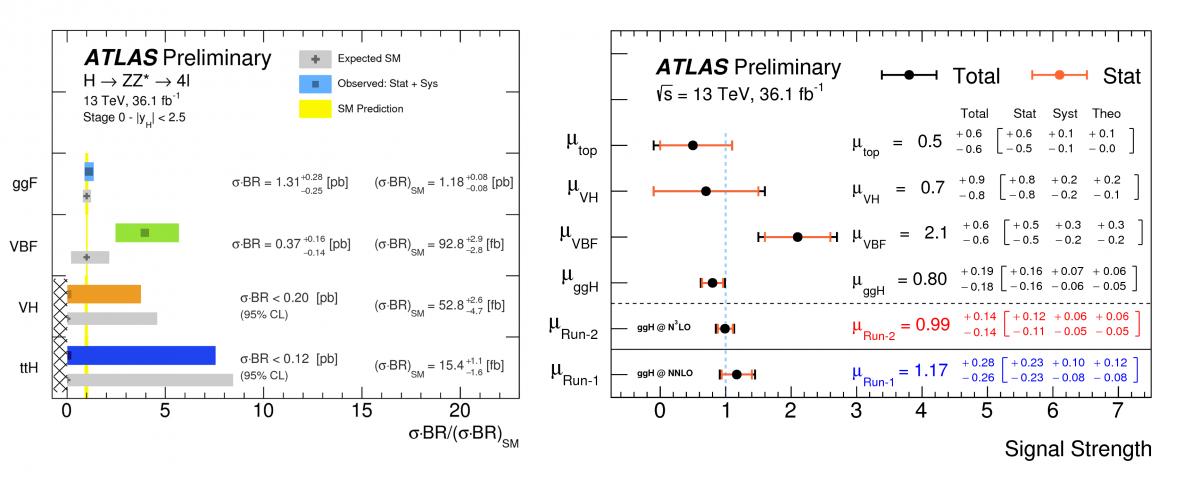Figures 1 and 2: Measurement of the Higgs boson production cross sections in its main production modes and normalised to the Standard Model predictions, as obtained by the H→ZZ*→4ℓ and H→γγ decay channels respectively. (Image: ATLAS Collaboration/CERN)

Since resuming operation for Run 2, the LHC has been producing about 20,000 Higgs bosons per day in its 13 TeV proton–proton collisions. At the end of 2015, the data collected by the ATLAS and CMS collaborations were already enough to re-observe the Higgs boson at the new collision energy. Now, having recorded more than 36,000 trillion collisions between 2015 and 2016, ATLAS can perform ever more precise measurements of the properties of the Higgs boson.

Measuring how the Higgs boson is produced and it decays is one of the major goals of the LHC experiments. Greater precision in these measurements allows us to refine our understanding of the Higgs sector of the Standard Model, and also constrain new phenomena beyond the Standard Model that would modify the coupling of the Higgs with the other Standard Model particles. By studying the Higgs boson decays to photon pairs (H→γγ) and to four leptons via intermediate Z bosons (H→ZZ*→4ℓ, where the ‘*’ indicates that one Z boson is produced off its mass shell), the ATLAS experiment can measure the coupling properties of the Higgs boson with unprecedented precision.

At the LHC, the Higgs boson is produced through different processes with very different rates: gluon fusion, vector-boson fusion, WH, ZH, and ttH. To probe these production modes, ATLAS has introduced a set of criteria to categorize the Higgs events with the H→γγ and H→ZZ*→4ℓ final states. The results of this study are displayed in Figures 1 and 2, where the measured cross section, normalized to the value predicted by the Standard Model, is shown.

### Combining these separate measurements allowed ATLAS to bring the experimental sensitivity closer to the precision of the Standard Model predictions.

With the LHC producing an ever-increasing number of Higgs bosons, ATLAS has been able to start measuring the cross section of each production mode in different phase-spaces, setting an additional stress test for the Standard Model. These results are used to constrain possible modifications of the Higgs boson couplings from those predicted by the Standard Model. No significant deviation from the prediction has yet been observed.

The H→γγ decay channel is also used to measure several differential cross sections for observables sensitive to Higgs boson production and decay, where good agreement was found between the data and Standard Model predictions (see Figure 4). Similar measurements have already been performed with H→ZZ∗→4ℓ decays.

Combining these separate measurements allowed ATLAS to bring the experimental sensitivity closer to the precision of the Standard Model predictions. The total Higgs boson production cross section is measured to be 57.0  +6.0−5.9  +3.2−2.7 pb, where the first uncertainty is statistical and the second of systematic origin. The result is consistent with the Standard Model prediction of 55.6 +2.4−3.4 pb. (Figure 3)

ATLAS will continue to study the Higgs boson properties for the rest of Run 2, isolating its rare production modes and measuring its more elusive properties. Uncovering these secrets will either further cement the Standard Model, or give us insight of what lies beyond.Figure 3: Total Higgs boson production cross sections measured at centre-of-mass energies of 7, 8, and 13 TeV by the H→γγ and H→ZZ*→4ℓ* channels and their combinations, compared to the Standard Model predictions. (Image: ATLAS Collaboration/CERN)Figure 4: Transverse momentum of the Higgs boson as measured in the Hyy decay, and compared to the Standard model predictions. (Image: ATLAS Collaboration/CERN)